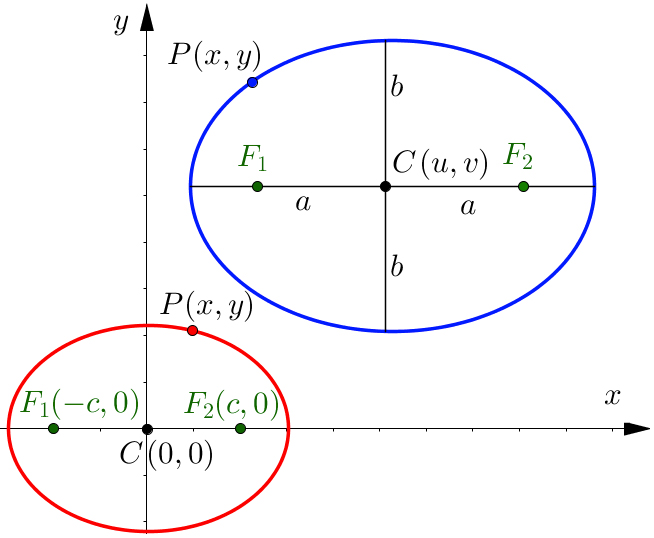Analytical Geometry 2D

# Ellipse

## Keywords: sllipseCentral Ellipse

$\frac{{x}^{2}}{{a}^{2}}+\frac{{y}^{2}}{{b}^{2}}=1$

Focal points of a Central Ellipse

${F}_{1}\left(-c,0\right);{F}_{2}\left(c,0\right);c=\sqrt{{a}^{2}-{b}^{2}}$

Non-Central Ellipses

$\frac{\left(x-u{\right)}^{2}}{{a}^{2}}+\frac{\left(y-v{\right)}^{2}}{{b}^{2}}=1$